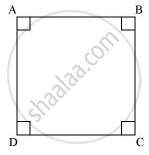Share

# Give a definition for square. Are there other terms that need to be defined first? What are they, and how might you define them? - CBSE Class 9 - Mathematics

ConceptEuclid’S Definitions, Axioms and Postulates

#### Question

Give a definition for square. Are there other terms that need to be defined first? What are they, and how might you define them?

#### Solution

Square :-

A square is a quadrilateral having all sides of equal length and all angles of same measure, i.e.,90° To define square, we must know about quadrilateral, side, and angle.Is there an error in this question or solution?

#### APPEARS IN

NCERT Solution for Mathematics Class 9 (2018 to Current)
Chapter 5: Introduction to Euclid's Geometry
Ex. 5.10 | Q: 2.5 | Page no. 85

#### Video TutorialsVIEW ALL 

Solution Give a definition for square. Are there other terms that need to be defined first? What are they, and how might you define them? Concept: Euclid’S Definitions, Axioms and Postulates.
S